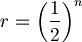# Coefficient of RelatednessThis article was checked by pedagogue, but more than year ago. Signature:  OSeda (talk)

 English: Coefficient of relatedness, coefficient of relationshipCzech: koeficient příbuznostiCoefficient of relatedness refers to probability that two related individuals have inherited a particular allele of the single locus / gene from their common ancestor (such allele is referred to as IBD - identical by descent). The coefficient is calculated as follows$r=\left(\frac{1}{2}\right)^n$

where n represents the number of steps in genealogy.